# 10.1 Formulas for Symmetries in Cartesian Coordinates

In the formulas below, a multiplication between a matrix and a triple of coordinates should be carried out regarding the triple as a column vector (or a matrix with three rows and one column).

Translation by (x,y,z):

(x,y,z)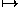(x+x, y+y, z+z)

Rotation through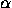(counterclockwise) around the line through the origin with direction cosines a, b, c (see):

(x,y,z)M(x,y,z),

where M is the matrix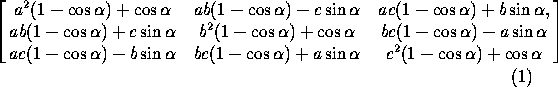Rotation through(counterclockwise) around the line with direction cosines a,b,c through an arbitrary point (x,y,z):

(x,y,z)(x,y,z)+M(x-x, y-y, z-z),

where M is given by (1) .

Arbitrary rotations and Euler angles. Any rotation of space fixing the origin can be decomposed as a rotation by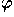about the z-axis, followed by a rotation by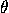about the y-axis, followed by rotation by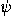about the z-axis. The numbers,andare called the Euler angles of the composite rotation, which acts as follows:

(x,y,z)M(x,y,z),

where M is the matrix given by(An alternative decomposition, more natural if we think of the coordinate system as a rigid trihedron that rotates in space, is the following: a rotation byabout the z-axis, followed by a rotation byabout the rotated y-axis, followed by a rotation byabout the rotated z-axis. Note that the order is reversed.)

Provided thatis not a multiple of 180°, the decomposition of a rotation in this form is unique (apart from the ambiguity arising from the possibility of adding a multiple of 360°to any angle). Figure 1 shows how the Euler angles can be read off geometrically.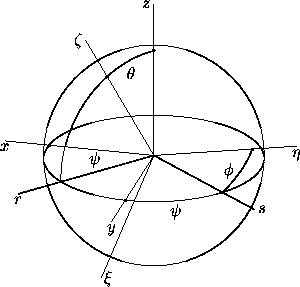Figure 1: The coordinate rays Ox, Oy, Oz, together with their images O, O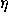, O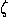under a rotation, fix the Euler angles associated with that rotation, as follows:=zO,=xOr=yOs, and=sO. (Here the ray Or is the projection of Oto the xy-plane. The ray Os is determined by the intersection of the xy- and-planes.)

Warning. Some references define Euler angles differently; the most common variation is that the second rotation is taken about the x-axis instead of about the y-axis.

Screw motion with angleand displacement d around the line with direction cosines a, b, c through an arbitrary point (x,y,z):

(x,y,z)(x+ad, y+bd, z+cd) + M(x-x, y-y, z-z),

where M is given by (1) .

Reflection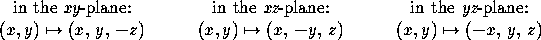Reflection in a plane with equation ax+by+cz+d=0: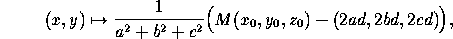where R is the matrix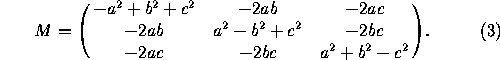Reflection in a plane going through (x,y,z) and whose normal has direction cosines a, b, c:

(x,y)(x+y+z)+M(x-x, y-y, z-z),

where M is as in (3) .

Glide-reflection in a plane P with displacement vector v: Apply first a reflection in P, then a translation by the vector v.

Next: 10.2 Formulas for Symmetries in Homogeneous Coordinates
Up: 10 Space Symmetries or Isometries
Previous: 10 Space Symmetries or IsometriesThe Geometry Center Home Page

Silvio Levy
Wed Oct 4 16:41:25 PDT 1995

This document is excerpted from the 30th Edition of the CRC Standard Mathematical Tables and Formulas (CRC Press). Unauthorized duplication is forbidden.